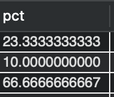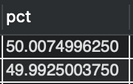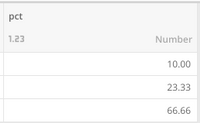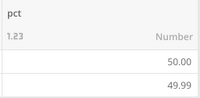# Precision Loss Redshift Data Flow

I am encountering a weird behaviour on using SQL SUM method on decimal values with DOMO Data Flow.

Expected result based on query from MySql:Result in DOMO after Redshift Data Flow completes:Issue is that precision is lost in this case and final result will be different in doing SUM on this values:

MySql => 100

DOMO => 99.99.

What can I do in this case to fix that part and to obtain same result as MySql?

•

You could utilize a ROUND(number, <decimal places>) function in a mysql dataflow to force it to do the rounding to the precision you want instead of having to truncate your numbers on input. If the percentage is calculated in your initial query you can use ROUND there instead of a MySQL data flow as well.

The other option if you must use magic ETL would be to use a calculator tile to multiply your percentage by 100 (shifting the decimal point over 2, use 1000 for 3 decimals of precision etc), set the data type to be a whole number (forces rounding) -> set the data type back to decimal -> calculator to divide by your original number (100 / 1000 etc).

• if possible, i would recommend against intentionally rounding metrics in ETL, keep the data as raw/accurate as possible.